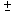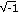# What Is a Number System?

## Understand the nature of the real number system, the elements and operations that make up the system, and some of the rules that govern the operations. Examine a finite number system that follows some (but not all) of the same rules, and then compare this system to the real number system. Use a number line to classify the numbers we use, and examine how the numbers and operations relate to one another.

In This Session
Part A:
A Simpler Number System
Part B: Comparing Number Systems
Part C: Building the Number Line
Homework

In this first session, you will use a finite number system and number lines to begin to gain a deeper understanding of the elements and operations that make up our infinite number system.

LEARNING OBJECTIVES:
In this session, you will do the following:

• Analyze a finite mathematical system
• Compare and contrast this system with the real number system
• Build a number line, from counting numbers to real numbers
• Find relationships between specific number sets on the number line and operations performed on other numbers on the number line
• Extend the number line to a coordinate system to represent complex numbers

### Notes

Note 1, Part A
If you are working in a group, discuss the different patterns you notice and why you think they occur. Here are some additional questions for discussion: What is meant by the terms “identity element” and “inverse element”? Can you see examples of commutative law in the table?

Note 2, Part C
If you are working in a group, discuss first how the number line gets filled up and why we need more numbers than just the counting numbers. This question will be revisited in the next session.

Note 3, Part C
Algebraic numbers also include some complex numbers (e.g.,, the solutions to x2 + 1 = 0). Such numbers, however, are excluded from this activity and this session since they cannot be represented on the number line.

### Key Terms

algebraic numbers

Algebraic numbers are all the numbers that are solutions to polynomial equations where the polynomials have rational coefficients (e.g. 1/2x3 – 3x2 + 17x + 5/8). They include all integers, rational numbers, and some irrational numbers (e.g.,, which are the solutions to x2 – 2 = 0). Note that algebraic numbers also include some complex numbers (e.g.,, the solutions to x2+ 1 = 0).

closed set

A closed set under a given operation is such that the result of the operation is always within the set.

complex numbers

Complex numbers are numbers composed by the addition of imaginary and real number elements. They are in the form a + bi, where a and b are real numbers, and i can be represented as i2 = -1 (a number such that when you square it, you get -1). Graphically, the complex numbers are typically shown as a plane, where the real part is represented horizontally and the imaginary part is represented vertically. The complex numbers are uncountably infinite, are closed under the four basic operations (other than dividing by 0), and have additive and multiplicative identity and inverses (other than 0). Additionally, the complex numbers are closed under any polynomial; that is, any polynomial with complex-number coefficients will have all its roots in the complex numbers.

counting numbers

Counting numbers are the same as natural numbers (i.e., 1, 2, 3, 4, …).

dense set

A dense set is such that for any two elements you choose, you can always find another element of the same type between the two. For example, integers are not dense; rational numbers are.

e

e is a transcendental number with the decimal approximation e = 2.7183. It is the base of natural logarithms. The value of e is found by taking the limit of (1 + 1/n)n as n approaches infinity. This number arises in many applications — for example, in calculus as a function whose value and slope are everywhere equal, and in compound interest as a base when interest is computed continuously.

even numbers

Even numbers are integers divisible by 2. Any number that ends with the digit 0, 2, 4, 6, or 8 is an even number.

identity element

If I is an identity element for operation *, then a * I = I * a = a for all elements a in the set. The identity element for addition of real numbers is 0, and the identity element for multiplication of real numbers is 1.

integers

Integers include positive and negative whole numbers, and 0.

inverse element

If b is the inverse element for a for operation *, then a * b = b * a = I, the identity element for that operation. The inverse for element a for addition is -a, because a + -a = -a + a = 0 for all values of a. The inverse for element a for multiplication is 1/a, because a * (1/a) = 1/a * a = 1 for all values of a except 0. Zero does not have an inverse for multiplication.

irrational numbers

Irrational numbers are numbers that can’t be expressed as a quotient of two integers, such as pi or square roots; they can only be expressed as infinite, non-repeating decimals.

odd numbers

Odd numbers are integers not divisible by 2. Any number that ends with the digit 1, 3, 5, 7, or 9 is an odd number.

perfect square

pi (or)

Pi (or) is a transcendental, irrational number that represents the ratio of circumference to diameter for every circle. The decimal approximation ofis 3.141593.

pure imaginary numbers

Pure imaginary numbers are numbers of the form b • i, where b is a real number and i is a number such that when squared yields -1 (i.e., i2 = -1). Graphically, the pure imaginary numbers are typically shown as a vertical line intersecting the number line at 0. They are uncountably infinite, closed under addition and subtraction only, and have additive identity and inverses.

rational numbers

Rational numbers are numbers that can be expressed as a quotient of two integers; when expressed in a decimal form they will either terminate (1/2 = 0.5) or repeat (1/3 = 0.333…)

real numbers

Real numbers comprise all rational and irrational numbers. They can be represented on a number line.

transcendental numbers

Transcendental numbers are numbers that cannot be the solution to a polynomial equation. The most common transcendental numbers areand e.

whole numbers

Whole numbers are the counting numbers and zero.